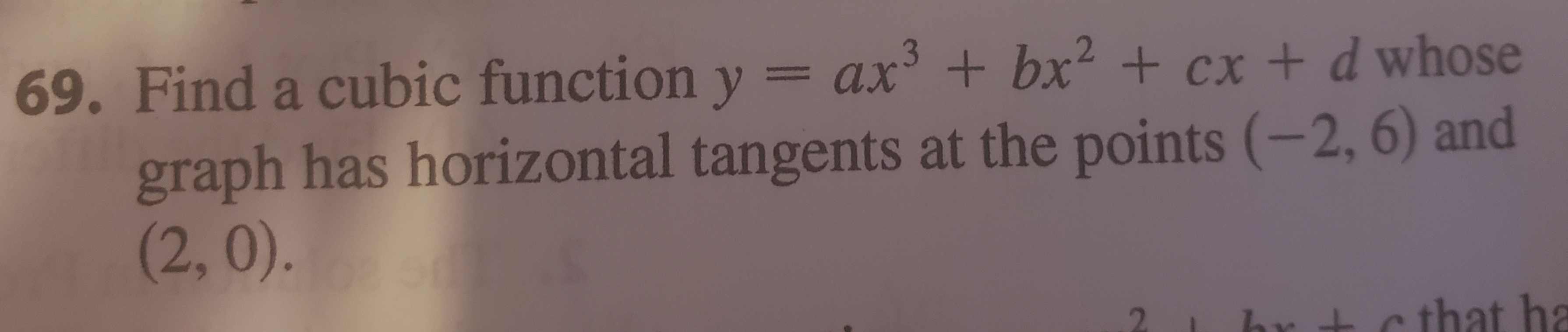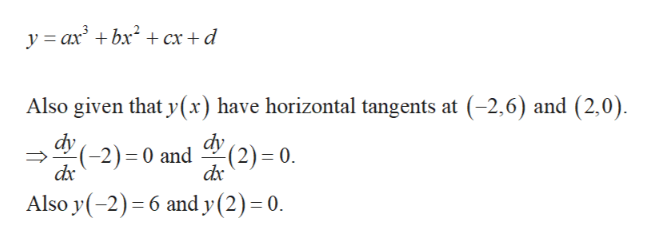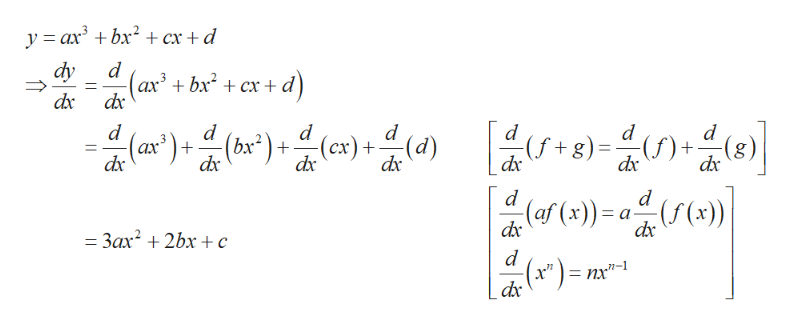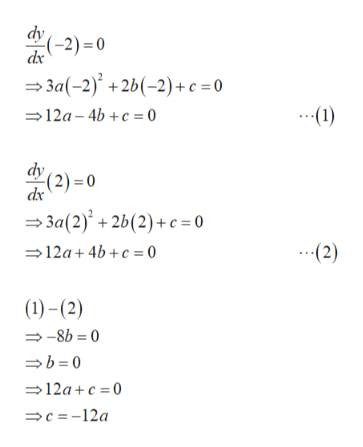# 69. Find a cubic function ygraph has horizontal tangents at the points (-2, 6) and(2, 0).= ax +bx +cx + d whosec that ha

Question
1 viewshelp_outlineImage Transcriptionclose69. Find a cubic function y graph has horizontal tangents at the points (-2, 6) and (2, 0). = ax +bx +cx + d whose c that ha fullscreen
check_circle

Step 1

The form of cubic function is given as follows.help_outlineImage Transcriptioncloseyax bxx +d Also given that y(x) have horizontal tangents at (-2,6) and (2,0) dy 2) = 0 dx dy2)-0 and Also y(-2) 6 and y (2) 0. fullscreen
Step 2

Obtain the values of unknown as follows.help_outlineImage Transcriptionclosey axbx cx + d dy d -(ax + bx2 + cx+ d dx 3 -띠 버음미음이 [ -9-습0금미 d d + dx d d d (ax) (bx*) (cx)+ dx dx dx dx dx dx d d (af (x)) a((x)) dx dx 3ax 2bx ¢ d nx"-1 fullscreen
Step 3

Simplify fur...help_outlineImage Transcriptionclosedy (-2) 0 dx 3a(-2)+2b(-2)+c =0 12a 4b+c o .1) dv -(2)= 0 dx 3a(2)+2b(2)+c= 0 12a 4b+c 0 (2) ()-(2) -8b 0 b0 12ac 0 c=-12a fullscreen

### Want to see the full answer?

See Solution

#### Want to see this answer and more?

Solutions are written by subject experts who are available 24/7. Questions are typically answered within 1 hour.*

See Solution
*Response times may vary by subject and question.
Tagged in

### Functions